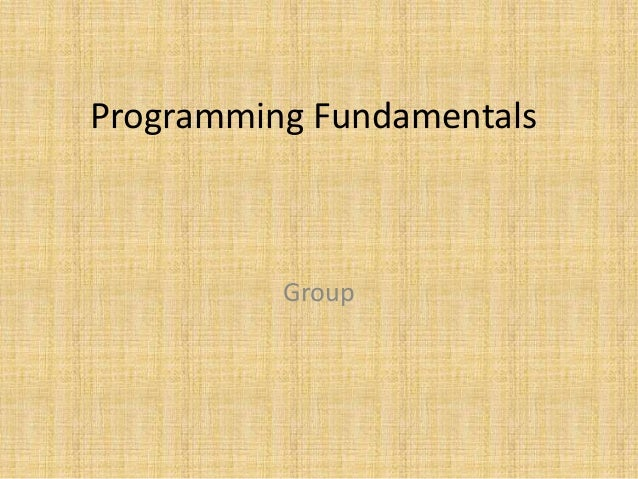Successfully reported this slideshow.Upcoming SlideShare
×

# structured programming

4,440 views

Published on

In this presentation you will learn about the "structured programming" in a very simple & easy way.

Published in: Software
• Full Name
Comment goes here.

Are you sure you want to Yes No• Be the first to comment

### structured programming

1. 1. Programming Fundamentals Group
2. 2. Structured Programming What is Structured Programming? In structured programming,we divide the whole program into small modules, so that program become easy to understand.
3. 3. Why we use Structured Programming? • We use structured programming because it enables the programmer to understand the program easily. • If a program consists of thousands of instructions and an error occurs than it is very difficult to find that error in the whole program but in structured programming we can easily detect the error and then go to that location and correct it. • This saves a lot of time.
4. 4. Structured Programming Concepts • Top Down Design. • Code reusability. • Information hiding.
5. 5. Function • We divide the whole program in to small blocks called functions. • Function is a block of statements that are executed to perform a task. • Function plays an important role in structured programming. • Using a function is like hiring a person to do a specific job.
6. 6. Function Call : • When a function is called by its name the control moves to the function definition and executes all the statements written in it • Semi colon is used after the name of function. Function Definition : • Function definition contains the instructions that are executed when a function is called • Semi colon is not used after the name of function.
7. 7. Function call and definition main() { message(); printf(“Hello world n”); } message() { printf(“Message function n”); } Waiting Function call Function definition
8. 8. Points to remember • C program must contains at least one function • Execution of C program begins with main() function. • If there are more than one function then one function must be main() • There is no limit on number of functions in C program • Functions in a program are called in sequence as mentioned in main() function • After the execution of function control returns to main() • A function can call itself such a process is called recursion.
9. 9. main() { printf("During Summer "); wakeup(); milk(); Study(); Play(); Sleep(); system("pause"); } study() { printf("I study at 2’pm"); } wakeup() { printf("I wake up at 6'am"); } milk() { printf("I drink milk at 8'am"); } Sleep() { printf("I sleep at 9'pm"); } Play() { printf("I play at 3'pm"); } Function Call Function Definition
10. 10. Function Prototype Function prototype contains following things about the function: • The data type returned by the function • The number of parameters received • The data types of the parameters • The order of the parameters • If there is no datatype and return value then we use void at the place of datatype and perameters
11. 11. int m(int,int); main() { int a,b,Mul; printf("Enter the values of a and b"); scanf("%d%d",&a,&b); Mul=m(a,b); printf("Multiplication of a & b is %d",Mul); system("pause"); } m(int x,int y) { int mul; mul=x*y; return(mul); } Function prototype Function Call Function Definition
12. 12. Imp Point • A function can return only one value at a time for example these statements are invalid: Return(a,b); Return(x,12);
13. 13. Return value Program int m(int,int); main() { int a,b,Mul; printf("Enter the values of a and b"); scanf("%d%d",&a,&b); Mul=m(a,b); printf("Multiplication of a & b is %d",Mul); system("pause"); } m(int x,int y) { int mul; mul=x*y; return(mul); }
14. 14. • If the value of a formal argument is changed in the called function, the corresponding change does not take place in the calling function. For example 30a 60b
15. 15. Variable Scope • Variable scope determine the area in a program where variable can be accessed. • When a variable loses its scope, it means its data value is lost • Common types of variables in C, – local – global • Global variable can be accessed any where in a program • Local variable can be accessed only in that function in which it is declared
16. 16. Example Program #include<stdio.h> int a,b; Global Variable main() { printf("Enter the values of a & b"); scanf("%d%d",&a,&b); sum(a,b); system("pause"); } sum(inta,intb) { int c; Local Variable c=a+b; printf("Sum is %d",c); }